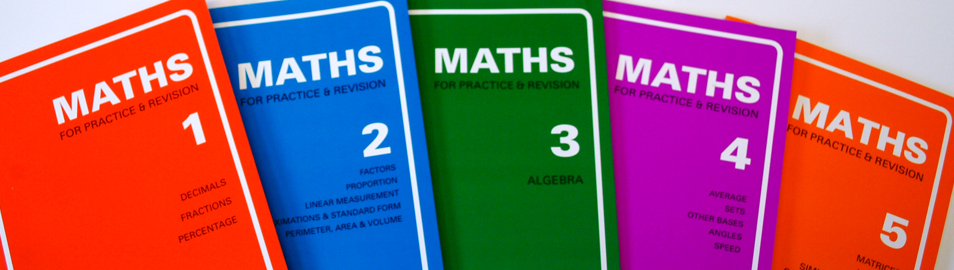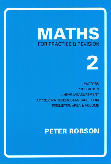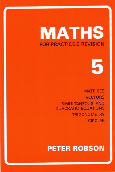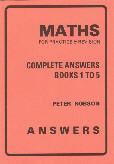A set of five books covering basic mathematical techniques up to Common Entrance, public school scholarship and GCSE.

Each double-page spread features a separate topic. The left hand page explains the method(s) involved, together with worked examples, and the right hand page gives related questions for the student to try. Thus the student requires little or no help from the teacher, making the series particularly useful for revision at home.

At the end of each book there are fifty mixed questions of the types found on earlier pages.

There is also a separate book which gives the answers to all the questions in the series.# Book 1

Price £3.95.

Decimal columns; four rules of decimals; converting decimals to fractions and vice versa; arranging decimals and fractions in order of size; percentage.

Suitable for students aged 9 and over.

A5 size (210 x 148 mm). 64 pages. Laminated red card covers.

ISBN 978-1-872686-21-9.# Book 2

Price £3.95.

Prime factors; HCF and LCM; squares and square roots; unitary sums; ratio; linear measurement; area and volume; estimation and approximation; standard form.

Suitable for students aged 9 and over.

A5 size (210 x 148 mm). 64 pages. Laminated blue card covers.

ISBN 978-1-872686-09-7.# Book 3 (Algebra)

Price £3.95.

Directed numbers; four rules of algebra; brackets; simple factorisation; substitution; equations and inequalities; formulae.

Suitable for students aged 9 and over.

A5 size (210 x 148 mm). 64 pages. Laminated green card covers.

ISBN 978-1-872686-05-9.# Book 4

Price £3.95.

24-hour clock; time problems; set notation and Venn diagrams; distance, speed and time; bases other than 10; angles, parallel lines; polygons.

Suitable for students aged 9 and over.

A5 size (210 x 148 mm). 72 pages. Laminated purple card covers.

ISBN 978-1-872686-02-8.# Book 5

Price £3.95.

Pythagoras' theorem; simultaneous equations; matrices; vectors; multiplication of two brackets; quadratic equations; probability; average (mean, mode, median and range); trigonometry; circles; solid figures. Includes square roots tables and sine, cosine and tangent table.

Suitable for students aged 11 and over.

A5 size (210 x 148 mm). 72 pages. Laminated orange card covers.

ISBN 978-1-872686-00-4.Price £4.95.

The answers to all the questions in Maths for Practice & Revision Books 1 to 5.

A5 size (210 x 148 mm). 116 pages. Salmon card covers.

ISBN 978-1-872686-17-2.GH Smith and Son The Advertiser Office, Market Place, Easingwold, York, YO61 3AB | 01347 821329 | info@ghsmith.com